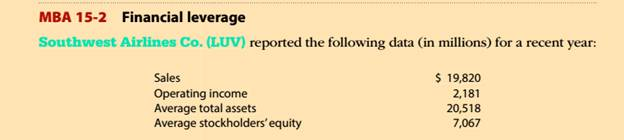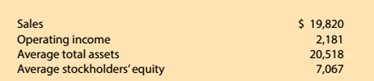Chapter 15, Problem 15.2.3MBA

Chapter
Section
Textbook ProblemFinancial leverageSouthwest Airlines Co. (LUV) the following data (in millions) for a recent year:Compute the profit margin, asset turnover, and financial leverage metrics using the expandedDuPont formula. Round profit margin, asset turnover, and financial leverage to two decimalplaces.Round return on stockholders’ equity to one decimal place.

To determine

Concept Introduction:

DuPont analysis is an Analysis to examine the Return of Equity (ROE) of a company by various means which includes Profit margin, Asset turnover and financial leverage.

To Calculate:

1. Profit margin
2. Investment turnover
3. Return on investment
Explanation

Statement showing calculation of Profit Margin, Investment Turnover and Return on Investment:

 Operating Income (A) \$ 2,181 Sales (B) \$ 19,820 Profit Margin (C) = A/B = 11...

Still sussing out bartleby?

Check out a sample textbook solution.

See a sample solution

The Solution to Your Study Problems

Bartleby provides explanations to thousands of textbook problems written by our experts, many with advanced degrees!

Get Started

Find more solutions based on key concepts